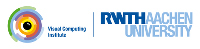OpenMesh
Extending the mesh using traits

This examples shows:

• How to extend the behaviour of entities using traits.

In the previous tutorial we used attributes and changed the type of the data types `Point`, `Normal`, `TexCoord`, and `Color`. But we can do even more with traits. We can change the behaviour of the mesh entities `Vertex`, `Face`, `Edge`, and `Halfedge`.

One goal in the design was a highly customizable data structure. Using the traits technique makes it possible. We pick up the smoother again and show an alternative way to implement it. Now we place the necessary data and the functions in the vertex itself

{
// store barycenter of neighbors in this member
{
private:
Point cog_;
public:
VertexT() : cog_( Point(0.0f, 0.0f, 0.0f ) ) { }
const Point& cog() const { return cog_; }
void set_cog(const Point& _p) { cog_ = _p; }
};

Note the definition of the vertex entity. We use the supplied define `VertexTraits` (which resolves in a rather inconvenient template definition). Similary we can use the defines `FaceTraits`, `EdgeTraits`, and `HalfedgeTraits` to extend these entities. Now we enhanced the vertex, with the additional member variable `cog_`, and the get/set-method pair to access the new member.

As before we compute in a first loop the barycenters for all vertices and store the information at the vertices

mesh.data(*v_it).set_cog(cog / valence);

In the second pass we set the new position of each vertex

mesh.set_point( *v_it, mesh.data(*v_it).cog());

It looks neat, but on the other hand we can't remove the data anymore as we could do with properties! By using traits one creates a 'static' configuration, which can't be changed during runtime.

The complete source looks like this:

#include <iostream>
#include <vector>
// -------------------- OpenMesh
#include <OpenMesh/Core/IO/MeshIO.hh>
#include <OpenMesh/Core/Mesh/TriMesh_ArrayKernelT.hh>
{
// store barycenter of neighbors in this member
{
private:
Point cog_;
public:
VertexT() : cog_( Point(0.0f, 0.0f, 0.0f ) ) { }
const Point& cog() const { return cog_; }
void set_cog(const Point& _p) { cog_ = _p; }
};
};
// ---------------------------------------------------------------------------
#define SIZEOF( entity,b ) \
std::cout << _prefix << "size of " << #entity << ": " \
<< sizeof( entity ) << std::endl; \
b += sizeof( entity )
template <typename Mesh>
void print_size(const std::string& _prefix = "")
{
size_t total=0;
SIZEOF(typename Mesh::Vertex, total);
SIZEOF(typename Mesh::Halfedge, total);
SIZEOF(typename Mesh::Edge, total);
SIZEOF(typename Mesh::Face, total);
std::cout << _prefix << "total: " << total << std::endl;
}
#undef SIZEOF
// ---------------------------------------------------------------------------
int main(int argc, char **argv)
{
MyMesh mesh;
// check command line options
if (argc < 4 || argc > 5)
{
std::cerr << "Usage: " << argv << " [-s] #iterations infile outfile\n";
exit(1);
}
int idx=2;
// display size of entities of the enhanced and the default mesh type
// when commandline option '-s' has been used.
if (argc == 5)
{
if (std::string("-s")==argv[idx-1])
{
std::cout << "Enhanced mesh size statistics\n";
print_size<MyMesh>(" ");
std::cout << "Default mesh size statistics\n";
print_size<MyMesh2>(" ");
}
// else ignore!
++idx;
}
std::cout<< " Input mesh: " << argv[idx] << std::endl;
if ( ! OpenMesh::IO::read_mesh(mesh, argv[idx]) )
{
std::cerr << "Error: Cannot read mesh from " << argv[idx] << std::endl;
return 0;
}
// smoothing mesh argv times
MyMesh::VertexIter v_it, v_end(mesh.vertices_end());
MyMesh::Scalar valence;
unsigned int i, N(atoi(argv[idx-1]));
std::cout<< "Smooth mesh " << N << " times\n";
for (i=0; i < N; ++i)
{
for (v_it=mesh.vertices_begin(); v_it!=v_end; ++v_it)
{
cog = cog = cog = valence = 0.0;
for (vv_it=mesh.vv_iter(*v_it); vv_it.is_valid(); ++vv_it)
{
cog += mesh.point( *vv_it );
++valence;
}
mesh.data(*v_it).set_cog(cog / valence);
}
for (v_it=mesh.vertices_begin(); v_it!=v_end; ++v_it)
if (!mesh.is_boundary(*v_it))
mesh.set_point( *v_it, mesh.data(*v_it).cog());
}
// write mesh to stdout
std::cout<< "Output mesh: " << argv[idx+1] << std::endl;
if ( ! OpenMesh::IO::write_mesh(mesh, argv[idx+1]) )
{
std::cerr << "Error: cannot write mesh to " << argv[idx+1] << std::endl;
return 0;
}
return 1;
}

Project OpenMesh, ©  Computer Graphics Group, RWTH Aachen. Documentation generated using doxygen .